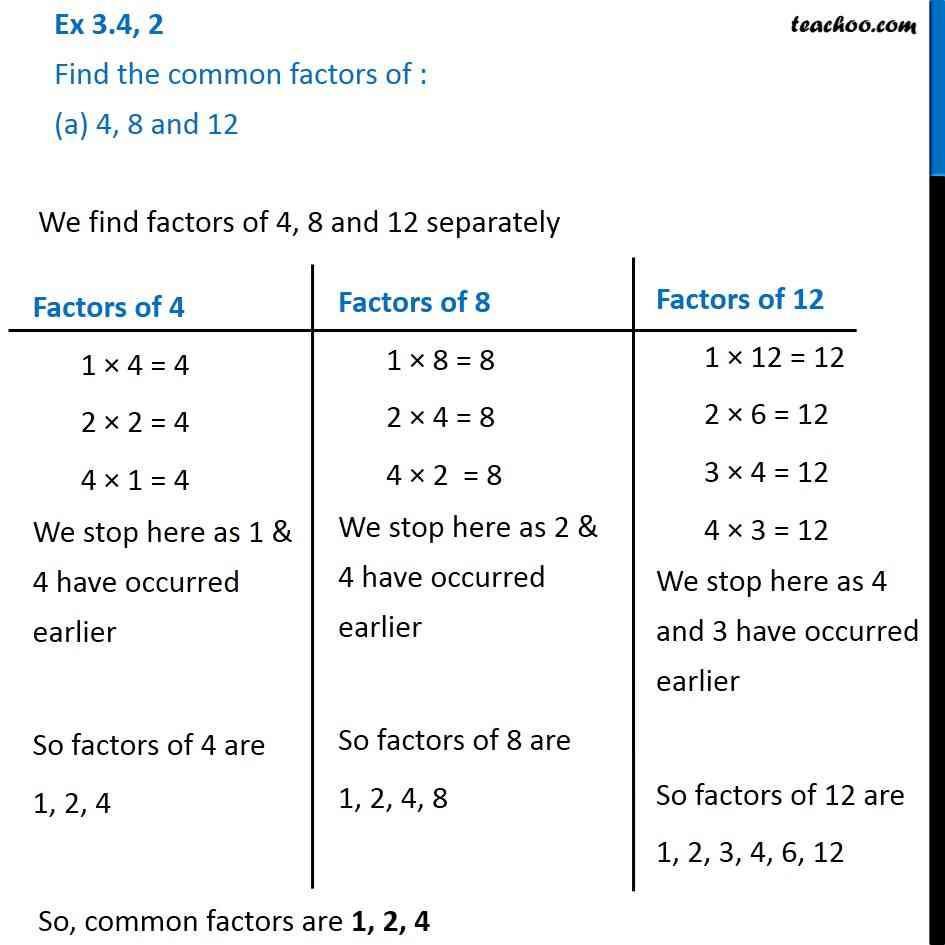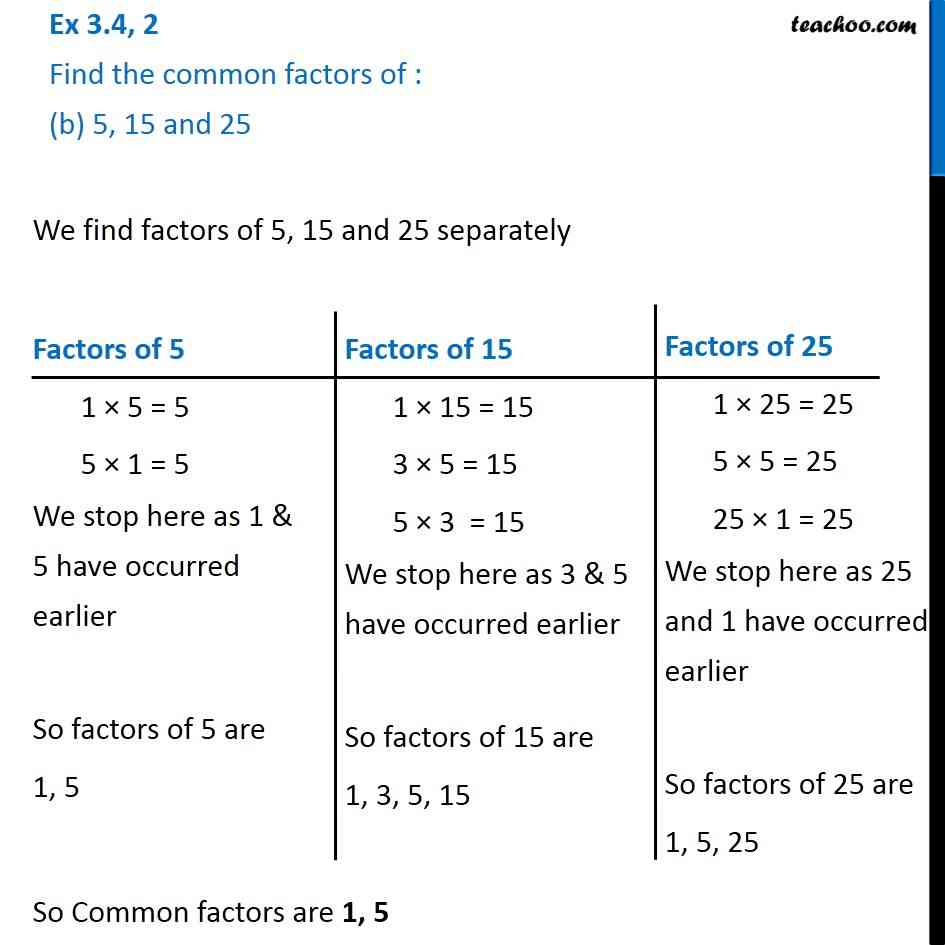1. Chapter 3 Class 6 Playing with Numbers
2. Concept wise
3. Common Factors

Transcript

Ex 3.4, 2 Find the common factors of : (a) 4, 8 and 12 We find factors of 4, 8 and 12 separately So, common factors are 1, 2, 4 Factors of 4 1 × 4 = 4 2 × 2 = 4 4 × 1 = 4 We stop here as 1 & 4 have occurred earlier So factors of 4 are 1, 2, 4 Factors of 8 1 × 8 = 8 2 × 4 = 8 4 × 2 = 8 We stop here as 2 & 4 have occurred earlier So factors of 8 are 1, 2, 4, 8 Factors of 12 1 × 12 = 12 2 × 6 = 12 3 × 4 = 12 4 × 3 = 12 We stop here as 4 and 3 have occurred earlier So factors of 12 are 1, 2, 3, 4, 6, 12 Ex 3.4, 2 Find the common factors of : (b) 5, 15 and 25 We find factors of 5, 15 and 25 separately So Common factors are 1, 5 Factors of 5 1 × 5 = 5 5 × 1 = 5 We stop here as 1 & 5 have occurred earlier So factors of 5 are 1, 5 Factors of 15 1 × 15 = 15 3 × 5 = 15 5 × 3 = 15 We stop here as 3 & 5 have occurred earlier So factors of 15 are 1, 3, 5, 15 Factors of 25 1 × 25 = 25 5 × 5 = 25 25 × 1 = 25 We stop here as 25 and 1 have occurred earlier So factors of 25 are 1, 5, 25

Common Factors

About the AuthorDavneet Singh
Davneet Singh is a graduate from Indian Institute of Technology, Kanpur. He has been teaching from the past 10 years. He provides courses for Maths and Science at Teachoo.